# R语言画图—ggplot2学习笔记

昨天终于把会议投稿的论文完成了，今天开始奋战另一篇拖了两个多月的文章，突然发现我两个月之前在这篇文章中用Excel做的图是那么low（没有贬低Excel的意思，有些方面还是很实用的），然后就在想，干脆用R画图，听说会很高大上，希望会为文章增添色彩，于是~就有了这篇学习笔记！

第一次写学习笔记，不懂套路，也不懂如何构思，如何让使我的这篇笔记看起来更有条理，就是顺着我学习的步骤，一步一步的将它记录下来。

#折线图
library(gcookbook)
library(ggplot2)
#连续变量的情况
ggplot(BOD, aes(x=Time, y=demand)) + geom_line()
#离散变量的情况
BOD1 <- BOD # 赋值数据
BOD1$Time <- factor(BOD1$Time)#变量因子化
ggplot(BOD1, aes(x=Time, y=demand, group=1)) + geom_line(linetype="dotted")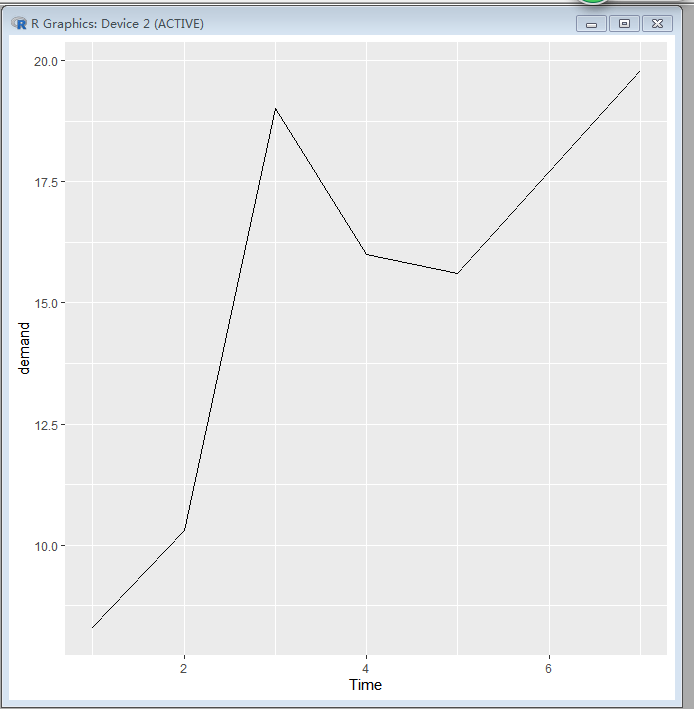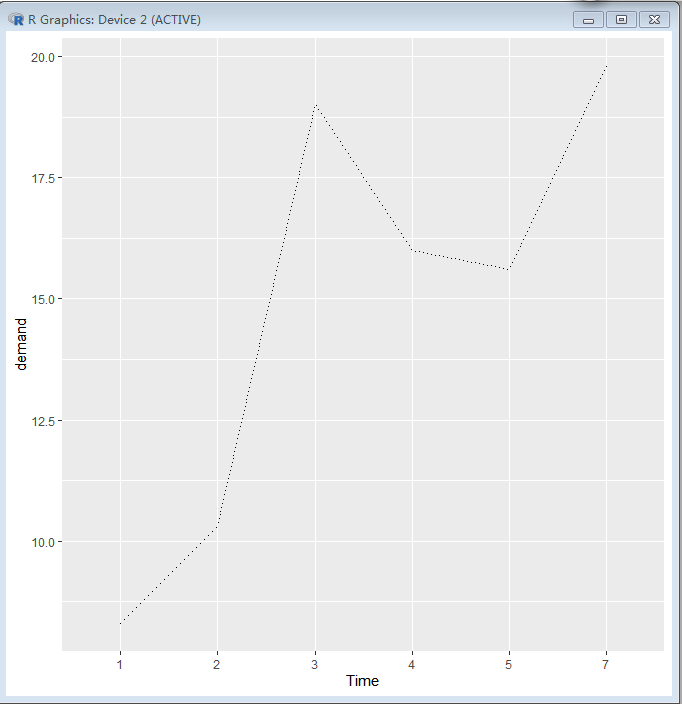#接下来试验自己的数据图
#工作空间
setwd("G:/单个性状"）#修改工作空间
#导入数据文件
summary(a)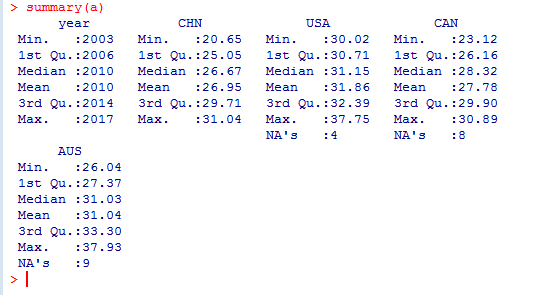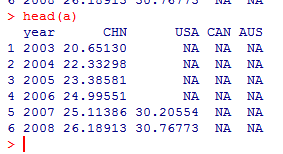ggplot(a, aes(x=year, y=CHN)) + geom_line()   #a为数据集名,x=,y=,分别是x轴和y轴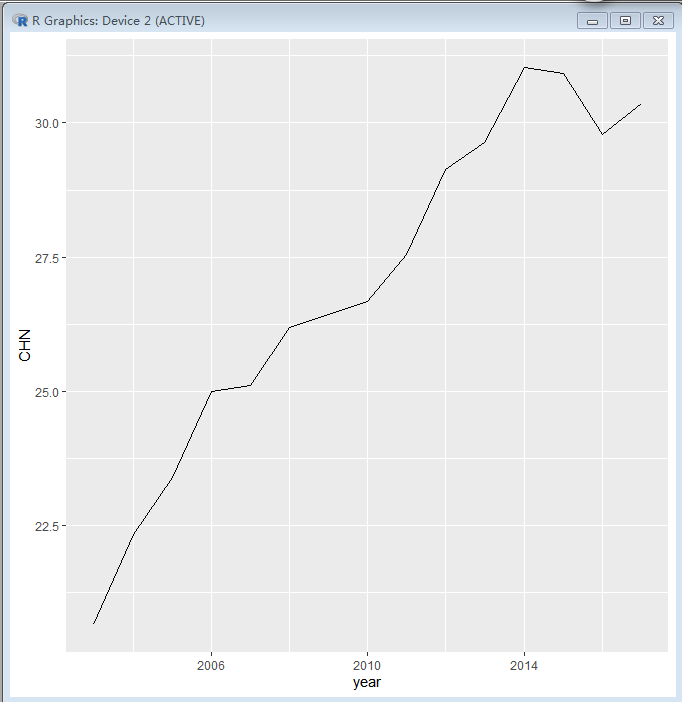ggplot(a, aes(x=year, y=CHN)) + geom_line() + geom_point(size=2, shape=17)

#geom_point是给折线图加上点，size和shape是定义点的大小和形状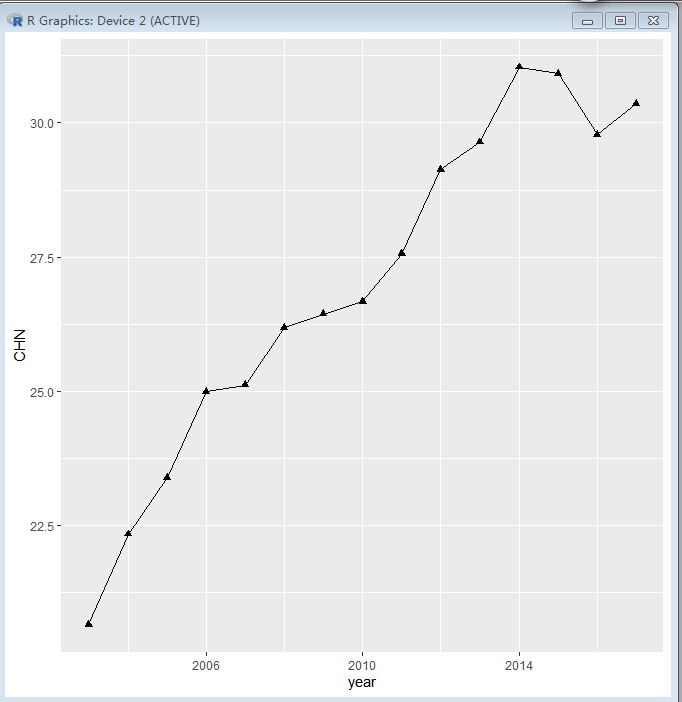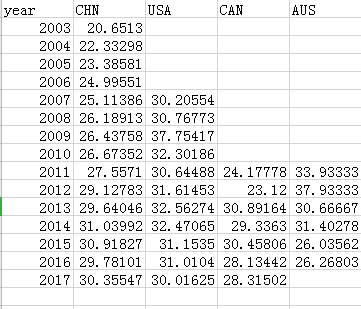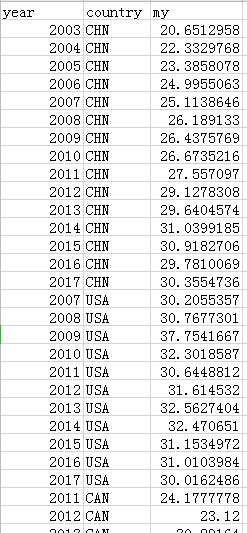ggplot(a, aes(x=year, y=my, colour=country,group=country))+geom_line(size=1)
#画的图线条太粗，没有美感、可以根据SIZE调节线条粗细ggplot(a,aes(x=year,y=my,color=country,shape=country))+geom_line()+geom_point(size=1)
#注意：geom_point修饰点的大小和形状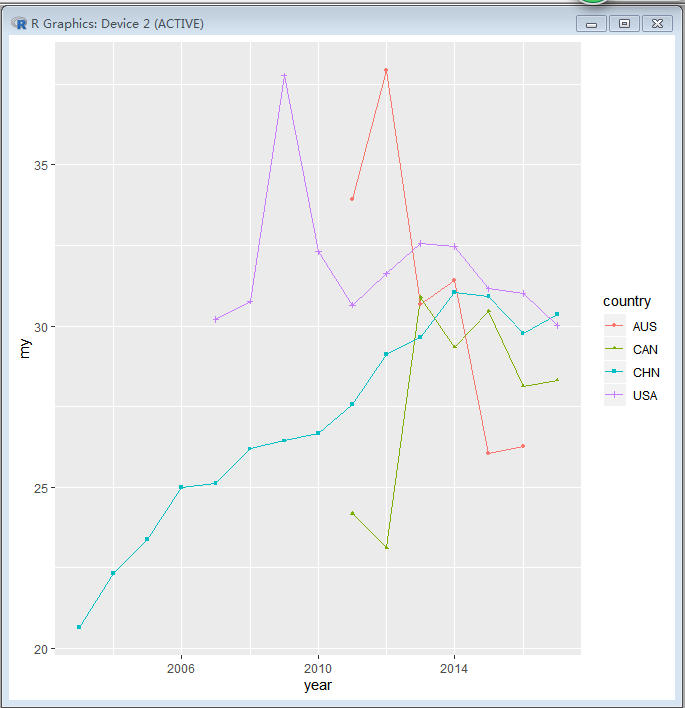ggplot(a,aes(x=year,y=my,color=country,fill=country))+geom_line()+geom_point(size=2)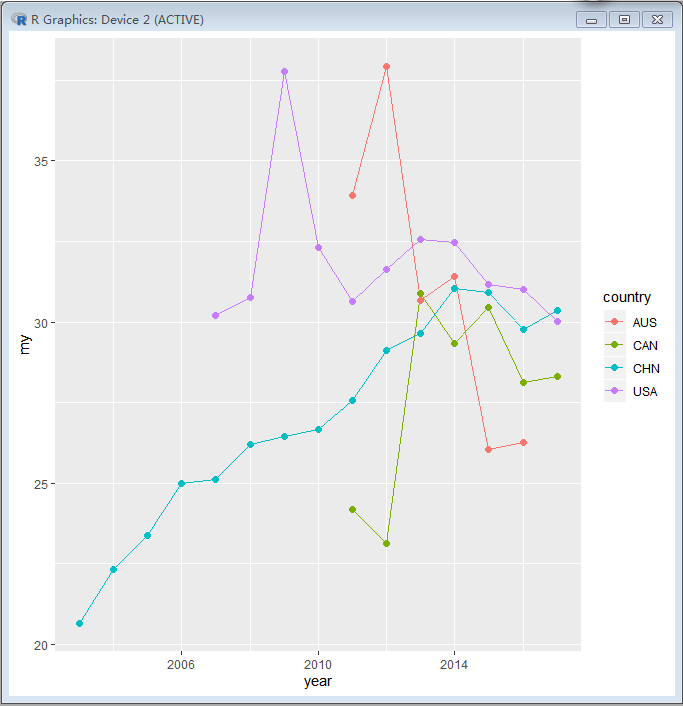xlab="",ylab="" #设置xy轴的轴标题

#单个变量分面、 依据facet_wrap()
ggplot(data = a) + geom_line(mapping = aes(x =Birthyear, y =my)) + facet_wrap(~country,nrow = 2)

#一个指标下几个水平下分面展示，nrow是分几行，ncol是分几列

#多个变量分面、依据 facet_grid()
ggplot(data = mpg) + geom_point(mapping = aes(x = displ, y = hwy)) + facet_grid(drv ~ cyl)  #不需要指定nrow和ncol

12-171175
06-095万+
01-011033
04-192451
07-012万+
12-271345
12-243754
07-13382
07-164万+
10-023590
11-261655
05-211万+
06-034687
12-313866
07-159642
08-15398
11-293094
08-313641
05-264663
02-18373

### “相关推荐”对你有帮助么？

•非常没帮助
•没帮助
•一般
•有帮助
•非常有帮助被折叠的  条评论 为什么被折叠?到【灌水乐园】发言¥2 ¥4 ¥6 ¥10 ¥20余额支付 (余额：-- )扫码支付获取中扫码支付点击重新获取扫码支付1.余额是钱包充值的虚拟货币，按照1:1的比例进行支付金额的抵扣。
2.余额无法直接购买下载，可以购买VIP、C币套餐、付费专栏及课程。余额充值